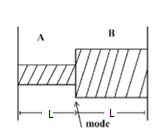The system of 2 rods shown in fig is vibrating at the same frequency and forming a standing wave. The ratio of the number of antinodes in the two rods if radius of rod B is twice the radius of A is:a.1

b.2

c.3

d.4

$$f_{1} = \frac{n}{2l}\sqrt{\frac{T}{\rho A}}f_{2} = \frac{m}{2l}\sqrt{\frac{T}{\rho 4A}}$$

Given f1 = f2

$$\frac{f_{1}}{f_{2}} = \frac{n}{m}\times 2$$ $$\frac{n}{m}\times 2$$(0)(0)